Latest Banking jobs   »

# Reasoning Ability Quiz For FCI Phase I 2022- 10th September

Directions (1-5): Read the following information carefully and answer the questions given below.
In a certain code language:
“Inside result happy spirit” is written as “ae me le ue”.
“Picture down morning inside” is written as “de le te ge”.
“Happy picture good spirit” is written as “te ae ue ce”.
“Spirit win morning spark” is written as “de ye we ae”.

Q1. What is the possible code for “good way down”?
(a) ce me ge
(b) pe me ge
(c) ce te le
(d) pe ce ge
(e) None of these

Q2. If “Inside win picture” is coded as “ye le te”, then what will be the code for “Spark”?
(a) xe
(b) we
(c) ce
(d) de
(e) Can’t determined

Q3. What is the code for “Morning”?
(a) de
(b) te
(c) ae
(d) ue
(e) None of these

Q4. What is the word for the code “me” in the given code language?
(a) spirit
(b) good
(c) result
(d) spark
(e) win

Q5. If “Spirit give happy” is written as “ue ae re”, then what will be the code for “Give”?
(a) ae
(b) ue
(c) re
(e) Can’t determine

Directions (6-10): Study the following number sequence and answer the questions following it.

8 1 3 2 5 4 7 6 8 9 6 1 3 5 2 8 4 4 4 5 2 7 8 2 9 7 2 1 5 6

Q6. How many even numbers are there in the numeric series which are immediately preceded by a number, which is a whole square?
(a) One
(b) Two
(c) Three
(d) More than three
(e) None of these

Q7. If all the odd numbers are dropped from the series, which number will be fifth to the right of seventh number from the left end?
(a) 2
(b) 8
(c) 6
(d) 4
(e) None of these

Q8. If all 1 is replaced by 2 and all 4 is replaced by 5 in the given number series, then which number will be fourth to the right of seventeenth number from the right end?
(a) 1
(b) 2
(c) 3
(d) 5
(e) 6

Q9. Which of the following number will be 8th to the right of 17th number from the right end?
(a) 5
(b) 6
(c) 8
(d) 9
(e) None of these

Q10. How many odd number/s which is/are immediately preceded by a ‘perfect square’ and immediately followed by a perfect cube are there in the above sequence?
(a) One
(b) Two
(c) Three
(d) More than three
(e) None

Q11. What should come in place of question mark (?) in the following series based on the above arrangement?
ZA YB XC WD ?
(a) EU
(b) LO
(c) LP
(d) VE
(e) None of these

Q12. How many numbers will be remained at the same position in the given number “46579739” when they are arranged in the ascending order from left to right?
(a) One
(b) Two
(c) Three
(d) More than three
(e) None of these.

Q13. If it is possible to make only one meaningful word with the 1st ,2nd ,4th and 7th letters of the word ‘CAREERPOWER’ which would be the third letter of the word from the right? If more than one such word can be formed give ‘Y’ as the answer. If no such word can be formed, give ‘Z’ as your answer.
(a) Y
(b) P
(c) C
(d) Z
(e) A

Q14. If 1 is added to each odd digit and 2 is subtracted from each even digit in the number 1436587, then how many digits will appear twice in the new number thus formed?
(a) Only 8
(b) Only 8 and 6
(c) 8, 6 and 4
(d) 2, 4 and 6
(e) None of these

Q15. How many letters will be remaining at the same position in the word ‘NIGHTKING’ when letters arranged according to alphabetical order from left to right?
(a) One
(b) Two
(c) Three
(d) More than Three
(e) None

Solutions

Solution (1-5):
Sol.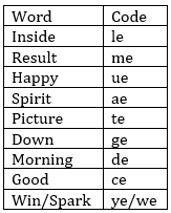S1. Ans. (d)
S2. Ans. (b)
S3. Ans. (a)
S4. Ans. (c)
S5. Ans. (c)

S6. Ans. (c)
Sol. 96, 44, 44
S7. Ans. (a)
S8. Ans. (d)
S9. Ans. (e)
S10. Ans. (e)

S11. Ans. (d)
Sol. VE

S12. Ans. (c)
Sol.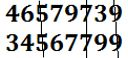S13. Ans. (a)
Sol. Pace, Cape

S14. Ans. (d)
Sol. 1436587
2244668

S15. Ans. (b)
Sol. NIGHTKING
GGHIIKNNT#### Congratulations!Download Hindu Review of October 2021: Free PDFIncorrect details? Fill the form again here

Download Hindu Review of October 2021: Free PDF

Thank You, Your details have been submitted we will get back to you.
•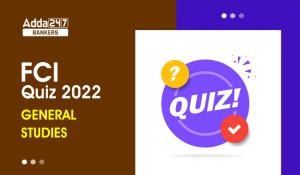General-Studies Quiz For FCI Phase I 202...
•World Teacher's Day 2022, Theme, History...
•Govt plans to set up 100 labs for 5G tec...
•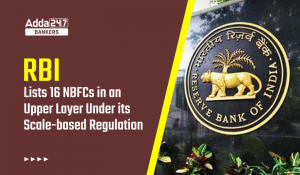RBI Lists 16 NBFCs in Upper Layer Under ...
•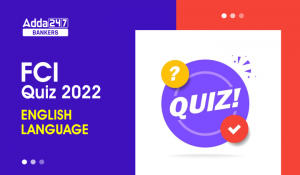English Quizzes For FCI Phase 1 2022- 3r...
•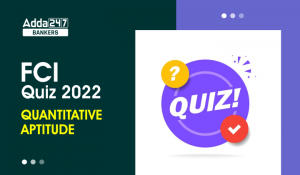Quantitative Aptitude Quiz For FCI Phase...